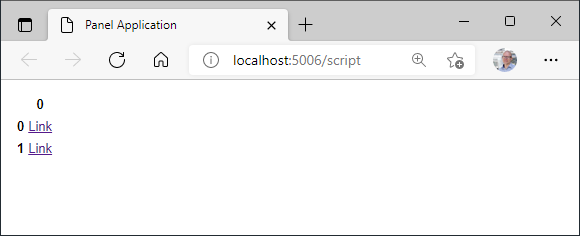# How do I include links in my DataFrame pane?

I’m asking for a friendHow would I include links/ urls in a table in Panel?

He’s tried something like

``````import panel as pn
import pandas as pd

pn.extension(sizing_mode="stretch_width")

def make_clickable(val):
return '<a href="{}">{}</a>'.format(val,val)

df.style.format(make_clickable)

table = pn.pane.DataFrame(df)

pn.Column(table).servable()
``````

But nothing happens when he click the links

Two things:

1. Even using pure pandas doing what you’re doing there has zero effect. `df.style.format` returns a new object, it does not modify the dataframe inplace (that only works on Tabulator).

2. We should support pandas `Styler` objects so you can do:

``````df = pd.DataFrame(['http://google.com', 'http://duckduckgo.com'])

def make_clickable(val):
return '<a href="{}">{}</a>'.format(val,val)

table = pn.pane.DataFrame(df.style.format(make_clickable))
``````

There’s a feature request for that here.

Thanks @philippjfr .

Looking at the implementation of `pn.pane.DataFrame` I found the `render_links`.

It does not solve the problem. But it helps a bit.

``````import panel as pn
import pandas as pd

pn.extension(sizing_mode="stretch_width")

pn.Column(table).servable()
``````

One temporary solution could be to use the DataFrame `styler` and the `HTML` pane.

``````import panel as pn
import pandas as pd

pn.extension(sizing_mode="stretch_width")

def make_clickable(val):

styler = df.style.format(make_clickable)
table = pn.pane.HTML(styler.to_html())

pn.Column(table).servable()
``````Another solution would be to create an improved `DataFrameX` pane.

``````from html import escape
import panel as pn
import pandas as pd
import param

pn.extension(sizing_mode="stretch_width")

# The urls depend on which row and column they are in
df = pd.DataFrame({
"country": ["DK", "DE"],
"1": ["<a href='https://www.google.com?country=DK&day=1' target='_blank'>1</a>", "<a href='?country=DE&day=1'>2</a>"],
"2": ["<a href='?country=DK&day=2'>3</a>", "<a href='?country=DE&day=2'>4</a>"]
})

class DataFrameX(pn.pane.DataFrame):
escape = param.Boolean(default=True)

_rerender_params = [*pn.pane.DataFrame._rerender_params, "escape"]

table = DataFrameX(df, escape=False)

pn.Column(table).servable()
``````

As far as I can see @philippjfr. The styler does not support adding links that depend on the row and column they are in. The `escape` parameter needs to be added to the `DataFrame` pane to support links.

I added a PR Add escape parameter to DataFrame pane to enable using html markup by MarcSkovMadsen · #2893 to support this use case.